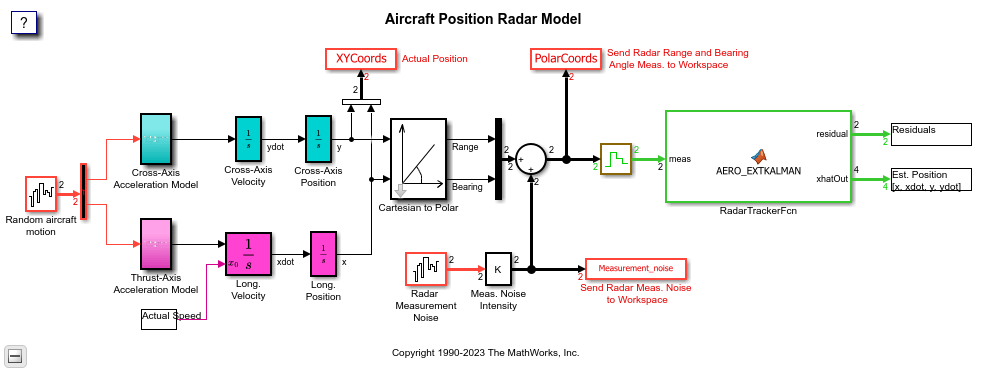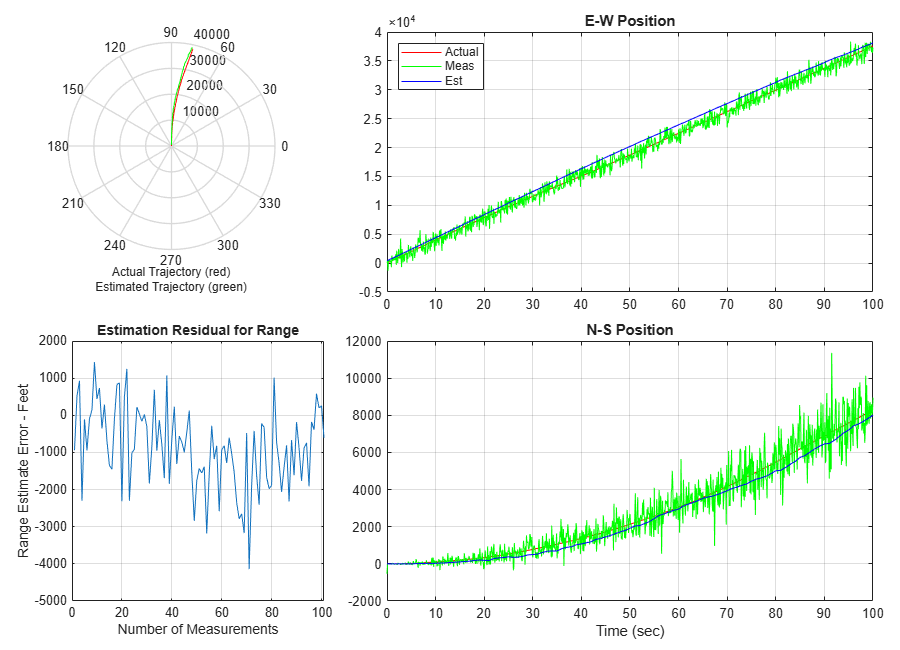This model shows the code generated for a Simulink® model containing a MATLAB® script.

The model contains an Extended Kalman Filter that estimates aircraft position from radar measurements. The MATLAB script rtwdemo_eml_aero_radar.m contains data for running the model. The estimated and actual positions are saved to the workspace and are plotted at the end of the simulation by the program rtwdemo_aero_radplot (called from the simulation automatically).

### Review and Simulate the Model

In this section you should review the model and perform a simulation.

```model='rtwdemo_eml_aero_radar'; open_system(model) rtwdemo_eml_aero_radar([],[],[],'compile'); rtwdemo_eml_aero_radar([],[],[],'term'); ```Open the MATLAB Function block `RadarTracker` in the MATLAB Editor.

```open_system([model,'/RadarTracker']) ```Simulate the model and review the results (displayed automatically).

```sim(model) ```### Generate Code for the Model

In this section you will generate code for the Kalman Filter portion of the model using the subsystem build functionality provided by Simulink Coder. In the first build, the model is configured to generate code using Simulink Coder™. In the second build, the model is configured to generate code using Embedded Coder®.

```% Create a temporary folder (in your system's temporary folder) for the % build and inspection process. currentDir = pwd; [~,cgDir] = rtwdemodir(); ```

Configure and build the model using Simulink Coder.

```rtwconfiguredemo(model,'GRT') slbuild([model,'/RadarTracker']) ```
```### Starting build procedure for: RadarTracker ### Successful completion of build procedure for: RadarTracker Build Summary Top model targets built: Model Action Rebuild Reason ============================================================================================= RadarTracker Code generated and compiled Code generation information file does not exist. 1 of 1 models built (0 models already up to date) Build duration: 0h 0m 19.709s ```

Configure and build the model using Embedded Coder.

```rtwconfiguredemo(model,'ERT') slbuild([model,'/RadarTracker']) ```
```### Starting build procedure for: RadarTracker ### Successful completion of build procedure for: RadarTracker Build Summary Top model targets built: Model Action Rebuild Reason ============================================================================================= RadarTracker Code generated and compiled Code generation information file does not exist. 1 of 1 models built (0 models already up to date) Build duration: 0h 0m 16.443s ```

A portion of `RadarTracker.c` is listed below.

```cfile = fullfile(cgDir,'RadarTracker_ert_rtw','RadarTracker.c'); rtwdemodbtype(cfile,'/* Model step', '/* Model initialize', 1, 0); ```
```/* Model step function */ void RadarTracker_step(void) { real_T P_d_tmp; real_T Phi_0; real_T Q; real_T Q_0; real_T M; real_T W; real_T tmp; real_T x_tmp; real_T Phi_1; real_T Bearinghat; real_T M_tmp; real_T M_tmp_0; real_T Rangehat; real_T r; real_T tmp_0; int32_T Phi_tmp; int32_T Phi_tmp_tmp; int32_T i; int32_T j; int8_T Phi; static const real_T e = { 0.0, 0.005, 0.0, 0.005 }; static const real_T c_b = { 90000.0, 0.0, 0.0, 1.0E-6 }; /* MATLAB Function: '<Root>/RadarTracker' incorporates: * Inport: '<Root>/meas' */ Phi = 1; Phi = 1; Phi = 0; Phi = 0; Phi = 0; Phi = 0; Phi = 1; Phi = 1; Phi = 0; Phi = 0; Phi = 1; Phi = 0; Phi = 0; Phi = 0; Phi = 0; Phi = 1; memset(&Q, 0, sizeof(real_T) << 4U); for (j = 0; j < 4; j++) { Q[j + (j << 2)] = e[j]; for (i = 0; i < 4; i++) { Phi_tmp_tmp = i << 2; Phi_tmp = j + Phi_tmp_tmp; Phi_0[Phi_tmp] = 0.0; Phi_0[Phi_tmp] += rtDW.P_d[Phi_tmp_tmp] * (real_T)Phi[j]; Phi_0[Phi_tmp] += rtDW.P_d[Phi_tmp_tmp + 1] * (real_T)Phi[j + 4]; Phi_0[Phi_tmp] += rtDW.P_d[Phi_tmp_tmp + 2] * (real_T)Phi[j + 8]; Phi_0[Phi_tmp] += rtDW.P_d[Phi_tmp_tmp + 3] * (real_T)Phi[j + 12]; } } for (i = 0; i < 4; i++) { Phi_1[i] = 0.0; for (j = 0; j < 4; j++) { Phi_tmp_tmp = (j << 2) + i; rtDW.P_d[Phi_tmp_tmp] = (((Phi_0[i + 4] * (real_T)Phi[j + 4] + Phi_0[i] * (real_T)Phi[j]) + Phi_0[i + 8] * (real_T)Phi[j + 8]) + Phi_0[i + 12] * (real_T)Phi[j + 12]) + Q[Phi_tmp_tmp]; Phi_1[i] += (real_T)Phi[Phi_tmp_tmp] * rtDW.xhat[j]; } } rtDW.xhat = Phi_1; rtDW.xhat = Phi_1; rtDW.xhat = Phi_1; rtDW.xhat = Phi_1; Rangehat = sqrt(rtDW.xhat * rtDW.xhat + rtDW.xhat * rtDW.xhat); Bearinghat = atan2(rtDW.xhat, rtDW.xhat); M_tmp = sin(Bearinghat); M_tmp_0 = cos(Bearinghat); M = M_tmp_0; M = 0.0; M = M_tmp; M = 0.0; M = -M_tmp / Rangehat; M = 0.0; M = M_tmp_0 / Rangehat; M = 0.0; rtY.residual = rtU.meas - Rangehat; rtY.residual = rtU.meas - Bearinghat; for (i = 0; i < 2; i++) { for (j = 0; j < 4; j++) { Phi_tmp_tmp = (j << 1) + i; x_tmp[j + (i << 2)] = M[Phi_tmp_tmp]; W[Phi_tmp_tmp] = 0.0; Phi_tmp = j << 2; W[Phi_tmp_tmp] += rtDW.P_d[Phi_tmp] * M[i]; W[Phi_tmp_tmp] += rtDW.P_d[Phi_tmp + 2] * M[i + 4]; } } for (i = 0; i < 2; i++) { for (j = 0; j < 2; j++) { Phi_tmp_tmp = j << 2; Phi_tmp = (j << 1) + i; Phi_1[Phi_tmp] = (((x_tmp[Phi_tmp_tmp + 1] * W[i + 2] + x_tmp[Phi_tmp_tmp] * W[i]) + x_tmp[Phi_tmp_tmp + 2] * W[i + 4]) + x_tmp[Phi_tmp_tmp + 3] * W[i + 6]) + c_b[Phi_tmp]; } } if (fabs(Phi_1) > fabs(Phi_1)) { r = Phi_1 / Phi_1; Rangehat = 1.0 / (r * Phi_1 - Phi_1); Bearinghat = Phi_1 / Phi_1 * Rangehat; M_tmp = -Rangehat; M_tmp_0 = -Phi_1 / Phi_1 * Rangehat; Rangehat *= r; } else { r = Phi_1 / Phi_1; Rangehat = 1.0 / (Phi_1 - r * Phi_1); Bearinghat = Phi_1 / Phi_1 * Rangehat; M_tmp = -r * Rangehat; M_tmp_0 = -Phi_1 / Phi_1 * Rangehat; } for (i = 0; i < 4; i++) { for (j = 0; j < 2; j++) { Phi_tmp_tmp = j << 2; Phi_tmp = i + Phi_tmp_tmp; tmp[Phi_tmp] = 0.0; tmp[Phi_tmp] += x_tmp[Phi_tmp_tmp] * rtDW.P_d[i]; tmp[Phi_tmp] += x_tmp[Phi_tmp_tmp + 1] * rtDW.P_d[i + 4]; tmp[Phi_tmp] += x_tmp[Phi_tmp_tmp + 2] * rtDW.P_d[i + 8]; tmp[Phi_tmp] += x_tmp[Phi_tmp_tmp + 3] * rtDW.P_d[i + 12]; } W[i] = 0.0; W[i] += tmp[i] * Bearinghat; r = tmp[i + 4]; W[i] += r * M_tmp; tmp_0 = W[i] * rtY.residual; W[i + 4] = 0.0; W[i + 4] += tmp[i] * M_tmp_0; W[i + 4] += r * Rangehat; rtDW.xhat[i] += W[i + 4] * rtY.residual + tmp_0; } for (i = 0; i < 16; i++) { Phi[i] = 0; } Phi = 1; Phi = 1; Phi = 1; Phi = 1; memset(&Q, 0, sizeof(real_T) << 4U); for (j = 0; j < 4; j++) { Q[j + (j << 2)] = 1.0; for (i = 0; i < 4; i++) { Phi_tmp_tmp = (i << 2) + j; P_d_tmp[Phi_tmp_tmp] = 0.0; Phi_tmp = i << 1; P_d_tmp[Phi_tmp_tmp] += M[Phi_tmp] * W[j]; P_d_tmp[Phi_tmp_tmp] += M[Phi_tmp + 1] * W[j + 4]; } } for (i = 0; i < 16; i++) { Q_0[i] = Q[i] - P_d_tmp[i]; } for (i = 0; i < 4; i++) { for (j = 0; j < 4; j++) { Phi_tmp_tmp = j << 2; Phi_tmp = i + Phi_tmp_tmp; Q[Phi_tmp] = 0.0; Q[Phi_tmp] += rtDW.P_d[Phi_tmp_tmp] * Q_0[i]; Q[Phi_tmp] += rtDW.P_d[Phi_tmp_tmp + 1] * Q_0[i + 4]; Q[Phi_tmp] += rtDW.P_d[Phi_tmp_tmp + 2] * Q_0[i + 8]; Q[Phi_tmp] += rtDW.P_d[Phi_tmp_tmp + 3] * Q_0[i + 12]; Phi_0[j + (i << 2)] = (real_T)Phi[Phi_tmp] - P_d_tmp[Phi_tmp]; } M[i] = 0.0; M[i] += W[i] * 90000.0; M[i + 4] = 0.0; M[i + 4] += W[i + 4] * 1.0E-6; } for (i = 0; i < 4; i++) { for (j = 0; j < 4; j++) { Phi_tmp_tmp = j << 2; Phi_tmp = i + Phi_tmp_tmp; Q_0[Phi_tmp] = 0.0; Q_0[Phi_tmp] += Phi_0[Phi_tmp_tmp] * Q[i]; Q_0[Phi_tmp] += Phi_0[Phi_tmp_tmp + 1] * Q[i + 4]; Q_0[Phi_tmp] += Phi_0[Phi_tmp_tmp + 2] * Q[i + 8]; Q_0[Phi_tmp] += Phi_0[Phi_tmp_tmp + 3] * Q[i + 12]; P_d_tmp[Phi_tmp] = 0.0; P_d_tmp[Phi_tmp] += M[i] * W[j]; P_d_tmp[Phi_tmp] += M[i + 4] * W[j + 4]; } } for (i = 0; i < 16; i++) { rtDW.P_d[i] = Q_0[i] + P_d_tmp[i]; } /* Outport: '<Root>/xhatOut' incorporates: * MATLAB Function: '<Root>/RadarTracker' */ rtY.xhatOut = rtDW.xhat; rtY.xhatOut = rtDW.xhat; rtY.xhatOut = rtDW.xhat; rtY.xhatOut = rtDW.xhat; } ```

You can view the entire generated code in a detailed HTML report, with bi-directional traceability between model and code.

```web(fullfile(cgDir,'RadarTracker_ert_rtw','html','index.html')) ```

Close the model and cleanup.

```bdclose(model) rtwdemoclean; cd(currentDir) ```